# What is a Binary Search Tree?

## Data Structures and Algorithm in C++

Originally Published at https://medium.com/swlh/binary-search-trees-c-484377f0d349

Let’s start with the simple definition of a Tree. Unlike linear data structures like Arrays and Linked Lists, trees are hierarchical data structures. It merely means data are stored as records which are connected to one another through different sorts of links. In most scenarios, trees are preferred more than a simple list because of its quick search as well as access feature. Feilds like Computer Programming and Networking utilize trees for various computational purposes like maintaining high bandwidths router algorithms for storing router tables and for data continuity in various programming libraries. There are many types of trees which implemented as per the specific purpose like Binary Trees, Binary Search Trees, Heaps and Balanced BST.

In the article, we will discuss Binary Search Trees, what are they, how they are implemented in a simple manner and why they are useful.

## Introduction

A binary search tree (BST) is a binary tree where each node has a Comparable key (and an associated value) and satisfies the restriction that the key in any node is larger than the keys in all nodes in that node’s left subtree and smaller than the keys in all nodes in that node’s right subtree (Sedgewick & Wyane, 2016)

BST is a sorted binary tree and also referred to as upside tree where it has root and leaves (aka node with no child). A Root is a topmost node also known as a parent node and a BST contains only one parent node. Each node must have at least at most two children who have left subtree and right subtree. These are also called as nodes of a BST. Nodes with at least one child become the parent of its node. And, a node with no children is called a leaf.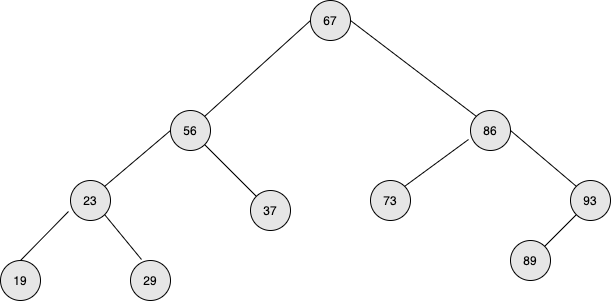There are three rules to be a BST:-

• The node’s left subtree contains only a key that’s smaller than the node’s Key.
• The node’s right subtree contains only a key that’s greater than the node’s Key.
• It cannot have a duplicate node’s key value.

A Binary Search Tree can be an unbalanced BST where it can either have left subtree or right subtree (skewed left or skewed right BST respectively). In skewed left BST, the right subtree is not present wherein skewed right BST; the left subtree is not present.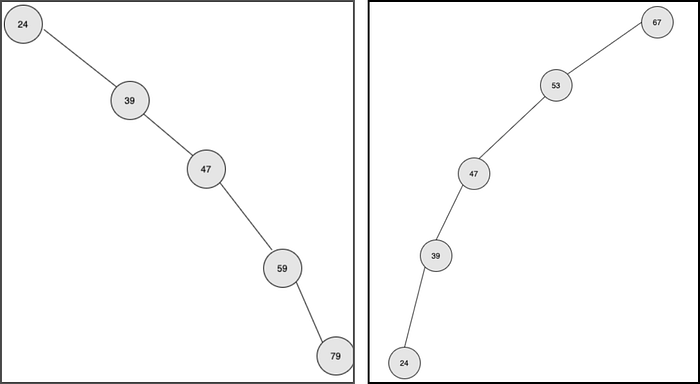After the short introduction to Binary Search Tree, let’s jump into the operations we can perform.

### ADT of Binary Search Tree

• `void insert(int key)`: Insert the node to the BST, and if there are no nodes in the BST, then it becomes the root node of the BST.
• `int PrintTreeInOrder()`: Print all the keys of BST sorted from Smallest key to the Greatest Key.
• `bool Search(int Key)`: Find the given Key in BST. If a key is found then return true if not return false.
• `int FindMin() and int FindMax()`: Find the minimum and maximum Key from BST.
• `int Successor(int key)` and `int Predecessor(int key)`: Find the successor and predecessor of a given key. I will get back to successor and predecessor later when I implement it.
• `void Remove(int key)`: Removes the given Key from BST.

So far we’ve covered an introduction to BST, types of BST, basic operations of a BST. Now, let’s jump into its implementation in C++.

### Implementation

In the first step in implementing BST, we will create a header file (BSTNode.hpp) where we define how our BST and BSTNode will look like and their corresponding functionalities. Basically it contains our Abstract Data Types of BST.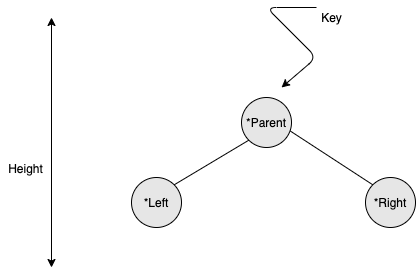### Code

``````//
//  BSTNode.hpp
//  BST
//
//  Created by Pramish Luitel on 12/5/20.
//

#ifndef BSTNode_hpp
#define BSTNode_hpp

#include <iostream>

class BSTNode
{
public:
int Key;
BSTNode * Left;
BSTNode * Right;
BSTNode * Parent;
int Height;
};

class BST
{
private:
BSTNode * root;

protected:
BSTNode * Insert(BSTNode * node, int key);
void PrintTreeInOrder(BSTNode * node);
BSTNode * Search(BSTNode * node, int key);
int FindMin(BSTNode * node);
int FindMax(BSTNode * node);
int Successor(BSTNode * node);
int Predecessor(BSTNode * node);
BSTNode * Remove(BSTNode * node, int v);

public:
BST();

void Insert(int key);
void PrintTreeInOrder();
bool Search(int key);
int FindMin();
int FindMax();
int Successor(int key);
int Predecessor(int key);
void Remove(int v);
};

#endif // BSTNODE_H``````

In the above class, we created two classes, i.e. BSTNode and BST class. BSTNode class holds the Left node, Right node, Parent node, Key and it’s Height which is self-explanatory. BST class holds all the available operations we will perform for our BST.

After creating the interfaces for our BST, we will create a BSTNode.cpp file to add our operations we are going to perform for BST. Let’s start with our first operation, i.e. Insert() operation.

### Insert()

Inserting a key to the BST is not as hard as we see Tree in a diagram. Each time we want to insert a key, we first have to compare it with the root node. If we do not have a root node, the initial Key will be the root node. If the root node is present then we compare the root node key with the inserted Key, if the Key is higher than the parent node, insert the Key to the right sub-tree and if the Key is smaller than the parent node insert the Key to the left sub-tree. We insert Key until we do not get the child of the node, i.e. last element of a child.

### Code

``````BSTNode * BST::Insert(BSTNode * node, int key)
{
// If BST doesn't exist
// create a new node as root
// or it's reached when
// there's no any child node
// so we can insert a new node here
if(node == NULL)
{
node = new BSTNode;
node->Key = key;
node->Left = NULL;
node->Right = NULL;
node->Parent = NULL;
}
// If the given key is greater than
// node's key then go to right subtree
else if(node->Key < key)
{
node->Right = Insert(node->Right, key);
node->Right->Parent = node;
}
// If the given key is smaller than
// node's key then go to left subtree
else
{
node->Left = Insert(node->Left, key);
node->Left->Parent = node;
}

return node;
}

void BST::Insert(int key)
{
// Invoking Insert() function
// and passing root node and given key
root = Insert(root, key);
}``````

Now, we inserted a key to the BST, which was pretty easy, right? Let’s start printing all the keys present in the tree which is PrintTreeInOrder() operation.

### PrintTreeInOrder()

This function prints all the keys in ascending order until all the keys are printed.

### Code

``````void BST::PrintTreeInOrder(BSTNode * node)
{
// Stop printing if no node found
if(node == NULL)
return;

// Get the smallest key first
// which is in the left subtree
PrintTreeInOrder(node->Left);

// Print the key
std::cout << node->Key << " ";

// Continue to the greatest key
// which is in the right subtree
PrintTreeInOrder(node->Right);
}

void BST::PrintTreeInOrder()
{
// Traverse the BST
// from root node
// then print all keys
PrintTreeInOrder(root);
std::cout << std::endl;
}``````

Now, we’ve printed all the keys in ascending order from a BST. Let’s start searching for a key in a BST which is Search() operation.

### Search()

We compare the given Key to the current node’s Key. If the Key is smaller than the current node’s Key, we go the left subtree; otherwise, we go to the right subtree. We do this until we do not find the Key.

### Code

``````BSTNode * BST::Search(BSTNode * node, int key)
{
// The given key is
if (node == NULL)
return NULL;
// The given key is found
else if(node->Key == key)
return node;
// The given is greater than
// current node's key
else if(node->Key < key)
return Search(node->Right, key);
// The given is smaller than
// current node's key
else
return Search(node->Left, key);
}

bool BST::Search(int key)
{
// Invoking Search() operation
// and passing root node
BSTNode * result = Search(root, key);

// If key is found, returns TRUE
// otherwise returns FALSE
return result == NULL ?
false :
true;
}``````

We’ve searched for a key in a BST. Let’s start implementing Minimum and Maximum Key in a BST which is FindMin() and FindMax() operation.

### FindMin() and FindMax()

In a BST, the minimum value will be stored at the left-most node of a tree, and maximum value will be stored at the right-most node of a tree.

### Code

``````int BST::FindMin(BSTNode * node)
{
if(node == NULL)
return -1;
else if(node->Left == NULL)
return node->Key;
else
return FindMin(node->Left);
}

int BST::FindMin()
{
return FindMin(root);
}

int BST::FindMax(BSTNode * node)
{
if(node == NULL)
return -1;
else if(node->Right == NULL)
return node->Key;
else
return FindMax(node->Right);
}

int BST::FindMax()
{
return FindMax(root);
}``````

Let’s start implementing the successor of a given key in a BST.

### Successor()

Successor means the greater key compared to the original key. To be a successor of a given key, we have three cases to be considered.

Case 1: If k is the maximum number in the tree, then k does not have a successor since it returns -1.

Case 2: If k does not have right subtree, then we have to find the ancestor of k, and until we find the node n which is higher than k, it will be the successor of k.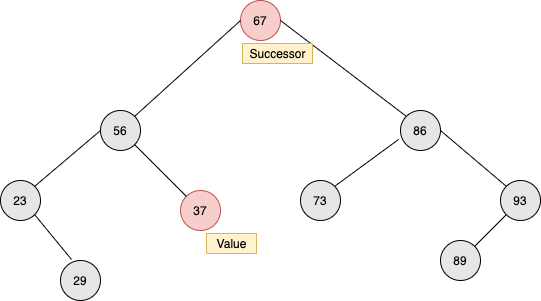Case 3: If k has the right subtree, the successor of k will be the smallest integer of the right subtree of k.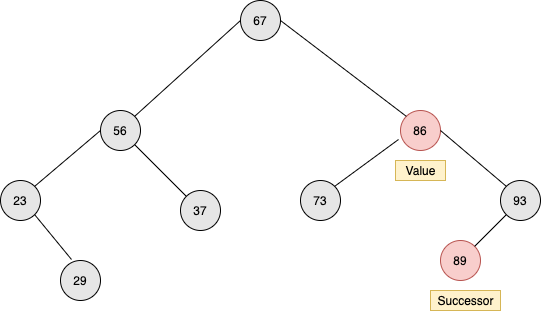### Code

``````int BST::Successor(BSTNode * node)
{
// The successor is the minimum key value
// of right subtree
if (node->Right != NULL)
{
return FindMin(node->Right);
}
// If no any right subtree
else
{
BSTNode * parentNode = node->Parent;
BSTNode * currentNode = node;

// If currentNode is not root and
// currentNode is its right children
// continue moving up
while ((parentNode != NULL) &&
(currentNode == parentNode->Right))
{
currentNode = parentNode;
parentNode = currentNode->Parent;
}

// If parentNode is not NULL
// then the key of parentNode is
// the successor of node
return parentNode == NULL ?
-1 :
parentNode->Key;
}
}

int BST::Successor(int key)
{
// Search the key's node first
BSTNode * keyNode = Search(root, key);

// Return the key.
// return -1
return keyNode == NULL ?
-1 :
Successor(keyNode);
}``````

Now we’ve implemented successor of a given key. Let’s start implementing the predecessor of a given key.

### Predecessor()

Predecessor means the smallest key compared to originally provided key. We also have three cases to be considered for getting the predecessor.

Case 1: If the given Key is minimum in a BST, then the Key does not have any predecessor.

Case 2: If the given Key does not have any left subtree, the predecessor of that Key will be the lower node which we get through traversing the ancestors of the Key.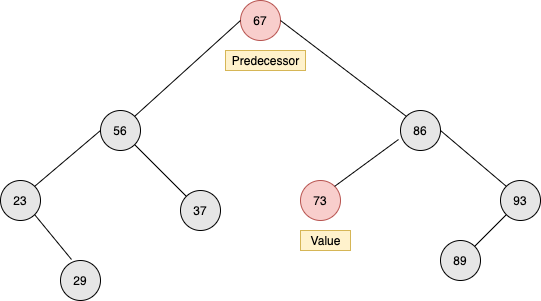Case 3: If the given Key has the left subtree, the predecessor of that Key will be the maximum integer of the left subtree of that Key.

I have to put the image here -> Image Link is not working

### Code

``````int BST::Predecessor(BSTNode * node)
{
// The predecessor is the maximum key value
// of left subtree
if (node->Left != NULL)
{
return FindMax(node->Left);
}
// If no any left subtree
else
{
BSTNode * parentNode = node->Parent;
BSTNode * currentNode = node;

// If currentNode is not root and
// currentNode is its left children
// continue moving up
while ((parentNode != NULL) &&
(currentNode == parentNode->Left))
{
currentNode = parentNode;
parentNode = currentNode->Parent;
}

// If parentNode is not NULL
// then the key of parentNode is
// the predecessor of node
return parentNode == NULL ?
-1 :
parentNode->Key;
}
}

int BST::Predecessor(int key)
{
// Search the key's node first
BSTNode * keyNode = Search(root, key);

// Return the key.
// return -1
return keyNode == NULL ?
-1 :
Predecessor(keyNode);
}``````

Now, we’ve implemented the predecessor of a given key. The last operation of a BST is to remove a key from BST and let’s apply it.

### Remove()

To remove the Key from a BST, we have to consider the following three cases.

Case 1: If the node does not have any child, then we can remove it and often called a leaf.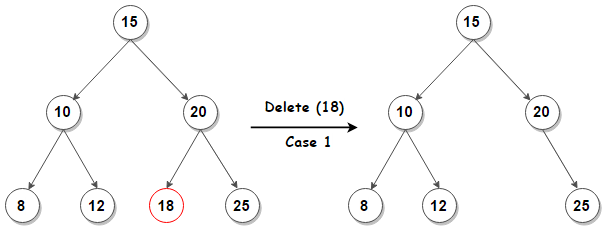Case 2: If want to remove the node that only has either Left or Right node, then we have to connect either the Left or Right node to the parent node and can only safely remove it.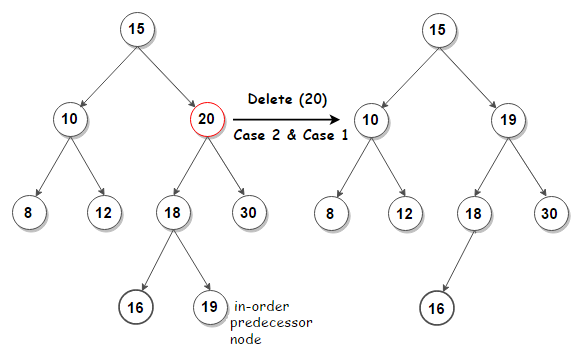Case 3: If we want to remove the node that has both Left and Right node then we have to determine the Successor (or Predecessor) and put that successor with the current node and then only remove that node.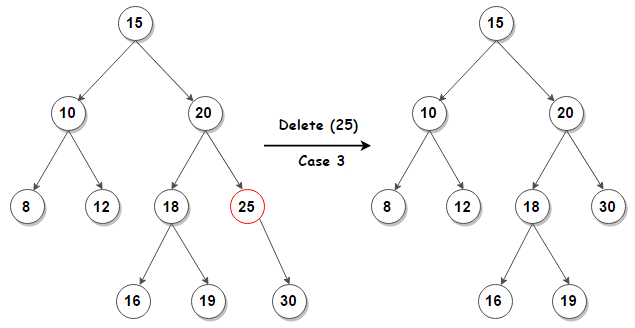### Code

``````BSTNode * BST::Remove(
BSTNode * node,
int key)
{
// The given node is
if (node == NULL)
return NULL;

// Target node is found
if (node->Key == key)
{
// If the node is a leaf node
// The node can be safely removed
if (node->Left == NULL && node->Right == NULL)
node = NULL;
// The node have only one child at right
else if (node->Left == NULL && node->Right != NULL)
{
// The only child will be connected to
// the parent's of node directly
node->Right->Parent = node->Parent;

// Bypass node
node = node->Right;
}
// The node have only one child at left
else if (node->Left != NULL && node->Right == NULL)
{
// The only child will be connected to
// the parent's of node directly
node->Left->Parent = node->Parent;

// Bypass node
node = node->Left;
}
// The node have two children (left and right)
else
{
// Find successor or predecessor to avoid quarrel
int successorKey = Successor(key);

// Replace node's key with successor's key
node->Key = successorKey;

// Delete the old successor's key
node->Right = Remove(node->Right, successorKey);
}
}
// Target node's key is smaller than
// the given key then search to right
else if (node->Key < key)
node->Right = Remove(node->Right, key);
// Target node's key is greater than
// the given key then search to left
else
node->Left = Remove(node->Left, key);

// Return the updated BST
return node;
}

void BST::Remove(int key)
{
root = Remove(root, key);
}``````

Finally, we’ve completed all the operations in a BST. It’s time to implement the main function where we can see all the operations we’ve defined above. We create a main.cpp file and ready to implement the main function and get to see the BST in action 😀

### main()

This is the starting point of a BST. We only implemented BSTNode.hpp file and we can now access the public functions of that file, i.e. BST() class.

### Code

``````//
//  main.cpp
//  main
//
//  Created by Pramish Luitel on 12/5/20.
//
#include <iostream>
#include "BSTNode.hpp"

using namespace std;

int main()
{
cout << "Binary Search Tree" << endl;

// Instantiate BST instance
BST * tree = new BST;

// Define key value to be inserted to BST
int keys[] = {23, 12, 31, 3, 15, 7, 29, 88, 53};

// Inserting keys
for(const int& key : keys)
tree->Insert(key);

// Traversing tree in order
// then print all keys
cout << "Tree keys: ";
tree->PrintTreeInOrder();

// Search key 31
// it should be found
cout << "Search key 31: ";
bool b = tree->Search(31);
if(b)
cout << "found";
else
cout << endl;

// Search key 18
// it should NOT be found
cout << "Search key 18: ";
b = tree->Search(18);
if(b)
cout << "found";
else
cout << endl;

// Retrieving minimum and maximum key
cout << "Min. Key : " << tree->FindMin();
cout << endl;
cout << "Max. Key : " << tree->FindMax();
cout << endl;

// Finding successor
// Successor(31) should be 53
// Successor(15) should be 23
// Successor(88) should be -1 or NULL
cout << "Successor(31) = ";
cout << tree->Successor(31) << endl;
cout << "Successor(15) = ";
cout << tree->Successor(15) << endl;
cout << "Successor(88) = ";
cout << tree->Successor(88) << endl;

// Finding predecessor
// Predecessor(12) should be 7
// Predecessor(29) should be 23
// Predecessor(3) should be -1 or NULL
cout << "Predecessor(12) = ";
cout << tree->Predecessor(12) << endl;
cout << "Predecessor(29) = ";
cout << tree->Predecessor(29) << endl;
cout << "Predecessor(3) = ";
cout << tree->Predecessor(3) << endl;

// Removing a key
cout << "Removing key 15" << endl;
tree->Remove(15);
cout << "Removing key 53" << endl;
tree->Remove(53);

// Printing all keys again
// Key 15 and 53 should be disappeared
cout << "Tree keys: ";
tree->PrintTreeInOrder();

return 0;
}``````

### Conclusion

We’ve implemented the complete Binary Search Tree using C++. In the next article, we will show how we can implement the Balanced Binary Search Tree where we have equal subtree in both left sub-tree and right sub-tree. The complete code to Binary Search Tree is https://github.com/pramish/Binary-Search-Tree.git. If you need further explanation or have any queries feel free to contact me or drop the responses below.

Stay Tuned guys. And, Have a good day and stay safe.